﻿ Experimental Determination of Planck’s constant using Light Emitting Diodes (LEDs) and Photoelectric Effect

Experimental Determination of Planck’s constant using Light Emitting Diodes (LEDs) and Photoelectric...

Andrea Checchetti, Alessandro Fantini

Experimental Determination of Planck’s constant using Light Emitting Diodes (LEDs) and Photoelectric Effect

Andrea Checchetti1,, Alessandro Fantini2

1Istituto d'Istruzione Superiore "Leonardo da Vinci"

2Liceo Scientifico "E. Fermi" Catanzaro Lido

Abstract

The aim of this experiment was to utilize the Inquiry Based Science Education, (IBSE) in order to offer the students a better understanding of the light-matter interaction. The inquiry-based teaching addresses the question of how the students make observations, develop hypotheses about phenomena, and devise tests to investigate their hypotheses. Using IBSE they share responsibility within the group for what concerns answering questions, and use a scientific approach to solve problems. 4th year students of undergraduate degree program of an Italian Arts Lyceum and 5th year students of undergraduate degree program of an Italian Scientific Lyceum have experimentally determined the value of Planck's constant, h. They have used respectively two methods: a) current-voltage measurements of a series of different colored LEDs; b) a method based upon the comparison of the incident light beams values of potential arrest with different wavelength on a photo emissive surface, using the photoelectric effect through an apparatus built on an optical bench. This work is an essential part of an apprenticeship developed during the “Professione Formatore in Didattica delle Scienze” Master, which takes place at the University of Calabria, with the authors taking part in it as trainees and that will be over in the month of October 2015.

• Andrea Checchetti, Alessandro Fantini. Experimental Determination of Planck’s constant using Light Emitting Diodes (LEDs) and Photoelectric Effect. World Journal of Chemical Education. Vol. 3, No. 4, 2015, pp 87-92. http://pubs.sciepub.com/wjce/3/4/2
• Checchetti, Andrea, and Alessandro Fantini. "Experimental Determination of Planck’s constant using Light Emitting Diodes (LEDs) and Photoelectric Effect." World Journal of Chemical Education 3.4 (2015): 87-92.
• Checchetti, A. , & Fantini, A. (2015). Experimental Determination of Planck’s constant using Light Emitting Diodes (LEDs) and Photoelectric Effect. World Journal of Chemical Education, 3(4), 87-92.
• Checchetti, Andrea, and Alessandro Fantini. "Experimental Determination of Planck’s constant using Light Emitting Diodes (LEDs) and Photoelectric Effect." World Journal of Chemical Education 3, no. 4 (2015): 87-92.

 Import into BibTeX Import into EndNote Import into RefMan Import into RefWorks

123
Prev Next

1. Introduction

The interaction of light and matter is synthetically expressed by Einstein-Planck equation: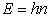(1)

where the constant of proportionality h has the following value: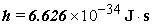It represents a milestone in the introduction to quantum mechanics; also known as “quantum of energy” or “quantum of action”. It is one of the fundamental physics constants, useful to understand the meaning of quantization in the atomic and nuclear world and represents the fundamental unit of action for discrete atomic scale system; it defines the minimum amount for the light energy and the electrons’ energies in atoms. Understand its physical meaning is the first step to bring students closer to the concept of quantization of energy, the starting point of a historical-didactic route that has as successive steps the wave nature of the electron, the uncertainty principle, the Schrödinger equation, until trace the development of the atomic structure. Light, quanta and Planck's constant are concepts that embrace both the chemistry that the physics.

The value of h was performed using Light Emitting Diodes (LEDs) and photoelectric effect.

In the Light Emitting Diodes (LEDs) method’s, we first make a complete circuit, using various apparatus (Figure 1). We use four colors (red, yellow, green and blue) for the different LEDs in the first place, to derive the voltage-current characteristic curves using the same circuit (Figure 3).

Then we use the linear part of voltage-current characteristic curves to estimate the slope (m) and intercept (n) corresponding to each LEDs (e.g., Figure 7 shows the red LED). These values are used to estimate Vg for different color LEDs, the potential that corresponds to the voltage for which the electrons are able to recombine with “holes” of the valence band. The Planck’s constant corresponding to each color is then determined using equation (3), whose values are presented in Table 2.

In the Photoelectric Effect method’s, light of a known frequency is allowed to fall on a metal photoemission surface acting as the photocathode (emitter plate in Fig. 6). Electrons are ejected from the cathode and some reach the collector producing a photoelectric current (Fig. 6).

The anode can be made negative (reverse bias) with respect to the cathode to repel the electrons. Only electrons leaving the emitter surface with initial kinetic energy greater than the potential energy between the cathode and collector will reach the anode and register a current. The potential difference can be increased until no electrons reach the collector and the current is stopped. This potential is called the stopping potential Va (eq. 6).

The above operation is repeated for two different radiations (incident light with differing frequencies) so that h can be estimated using equation 7.

The Planck’s constant value has been determined by using, under the educational profile, the same methodology: the Inquiry Based Science Education, (IBSE).

IBSE is a useful process that allows:

•  Creation or choice of activities that motivate and involve students

•  Determination of learning outcomes and appropriate assessment methods

•  Use of specialized tools and digital technology

•  Selection of educational resources, such as reading materials and useful websites

The teaching based on the research addresses the matter of how students make observations, how they develop hypotheses about the phenomena and how they can anticipate tests to investigate their hypothesis.

The inquiry process involved the following steps :

1) Engagement

2) Explore

3) Explain

4) Elaborate

5) Evaluate

For each of the experimental methods used by the authors to calculate the Planck constant, the following Learning Activities have been developed:

LIGHT EMITTING DIODES (LEDS) METHOD

1st Learning Activity (Developing the concept so that the students can gain an appreciation of the following)

The diode LED, as all the diodes, is a semiconductor device, which behaves as a bulb lighting up when connected to a voltage generator. The radiated energy can derive experimentally from the characteristic curve of the diode. Therefore, it can be easily seen that each LED is a "non-ohmic conductor", because the voltage-current relation does not follow Ohm's law of direct proportionality.

2nd Learning Activity (Hypothesis and Experiment Design)

When an electron and a hole meet, they recombine and generate a photon; electrical energy of the couple is transformed in the electromagnetic energy of the photon.

•  Discuss with the students about experiment design.

•  Identify the tools needed to get the current-voltage curves.

•  Building the circuit.

3rd Learning Activity (Experiment activity)

Measure the threshold voltage for different color LEDs:

•  Acquisition of the measures: varying the supply voltage of the circuit the students will measure the function of Vdiode.

•  To plot the trend of the current as a function of the potential difference.

•  Fit for the linear part of the curve of the four LEDs we used, I = mV - n. If I = 0 one obtains Vthreshold.

4th Learning Activity (Discussion and conclusion)

•  The students drawn the characteristic curve of dyed LEDs and using Excel determine the equation of the linear part;

•  With the calculation performed with Excel software, the students can determine the experimental value of Planck's constant.

•  For each value of h the student calculates the percentage error.

•  The light emission of the LED starts from a value of potential (Vg) that corresponds to the voltage for which the electrons are able to recombine with “holes” of the valence band. Experimentally, in order to determine the value of this potential the students proceed with an extrapolation of the linear part of the intensity curve of the current-voltage until it meets the x-axis.

•  The value given by the intersection represents the voltage value, which multiplied by the electron charge gives us the amount of the energy of the emitted photons.

•  Compare the experimental value of h with that of the literature.

PHOTOELECTRIC EFFECT METHOD

1st Learning Activity (Developing the concept in order that students can gain an appreciation of the following)

A Hg vapor lamp emits monochromatic light, exploiting the potential of an optical bench, passes through filters of different wavelength and strikes a photo emissive surface K. Also interesting is to note that the incident light is transformed into electricity.

2nd Learning Activity (Hypotheses and Experimental Design)

The current "emitted" from the photoemission surface can be suitably “braked” up to be stopped by a suitable contrary voltage (potential arrest), capable of preventing the electrons to reach the opposite polarity of the emissive device.

•  Discussing with the students on the modality of the of the apparatus structure.

•  Finding the best selection and sequence of filters, diaphragms and positioning in the optical bench.

•  Developing the experimental apparatus

3nd Learning Activity (experimental activity)

Measuring the potential stop for the different colored filters:

1. Development of the experience: changing the generator opposite voltage and bringing the index calibration of the micro-ammeter connected to the "0", it can be easily identified with the aid of a milliammeter Va.

2. Tracing the course of the current as a function of the potential difference.

4nd Learning Activity (discussion and conclusion)

1. Students draw the characteristic curve of the color filters and using Excel determines the equation of the linear part.

2. With the calculation made the students are able to determine the experimental value of Planck's constant.

3. For each value of h the student calculates the percentage of error.

The resetting of the micro ammeter is tied to the value of the potential (Va) which corresponds to the voltage for which the electrons are able to recombine with "holes” in the valence band. Experimentally, in order to determine the value of this potential, students proceed with a fine calibration of the generator, which is capable to reset the measure of the micro ammeter.

Then the experimental value of h is compared to the one present in the scientific literature.

2. Experimental Design

2.1. Principle

LIGHT EMITTING DIODES (LEDS) METHOD

One of the methods to measure the Planck constant is to derive the voltage-current characteristic curve for a series of different color LEDs.

The method uses the energy emitted when a photon is produced as shown in Figure 1Download asVeiw figureFigures index
Figure 1. The current -voltage curve for a typical LEDDownload asVeiw figureFigures index
Figure 2. The current -voltage curve for a typical LED

The diode LED, as all the diodes, is a semiconductor device , which behaves as a bulb lighting up when connected to a voltage generator. LED’s emit light only when the voltage is forward biased and above a minimum threshold value. Above the threshold, value the current increases exponentially with voltage. The radiated energy can derive experimentally from the characteristic curve of the diode. Therefore, we can observe that each LED is a "non-ohmic conductor", because the voltage-current relation does not follow Ohm's law of direct proportionality.

Figure 2 shows the characteristic curve of a LED. As can be seen from the figure up to a certain voltage value (V<Vg) the LED does not conduct, then for higher voltages (V>Vg) the LED begins to conduct and to emit light. To determine the value of Vg is necessary to make a linear fit of the linear part of the curve and calculate the point where this line intercepts the x-axis. We obtain a value of voltage (Vg) which, multiplied by the electron charge (e = 1.602x10-19 C), gives us precisely the energy of the emitted photons (Eg = eVg).

The equation of the linear part obtained using Excel is: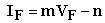(2)

With the calculation performed with Excel software, we can determine the experimental value of Planck's constant. Starting from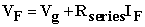from which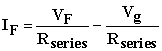And finally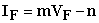Where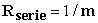And setting I = 0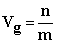Given that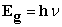And all the same time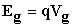we determine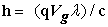(3)

Where we recognize that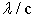is just the inverse of the frequency of the emitted photon, h is the Planck’s constant, q is the charge of the electron and Vg is the turn-off voltage of the LED .

PHOTOELECTRIC EFFECT METHOD

Using a particular device (photo emissive surface K), the interpretation of the photoelectric effect is based on a very simple and intuitive relation: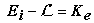(4)

where Ei is the energy of the incident photon, L the extraction work of the electron from the metal and Ke the kinetic energy of the electron released.

This relation, explaining the magnitudes, can be rewritten in the following way: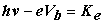(5)

At this point, by applying a d.d.p. as to have the polarities reversed: cathode → surface photoemission cathode and anode → collector, the electrons are clearly slowed and, acting on the micro ammeter potentiometer, you can go up to block the emitted electron flow (current). This d.d.p. takes the name of potential stop (Va), and then Eq. 4 becomes: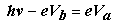(6)

If this operation was repeated for two different radiation accidents, the result would be: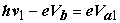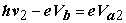and subtracting member to member: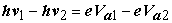up to obtain: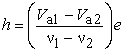(7)
2.2. Experimental Design

LIGHT EMITTING DIODES (LEDS) METHODDownload asVeiw figureFigures index
Figure 3. Apparatus for the measurements

The apparatus used in this experiment is shown in Figure 3 and is composed by:

•  Power supply

•  Diode bridge

•  LED system

•  Two Multimeters: one to be used as voltmeter and the other as an ammeter

The power supply is used to put in operation with the main power supply all equipment that cannot be connected directly to the 220V socket.

The diode bridge is used to convert an AC power signal into a signal in continuous energy.

The circuit that was used to experimentally determine Planck's constant is illustrated below (Figure 4):Download asVeiw figureFigures index
Figure 4. Electric circuit for measuring the voltage-current response of each LED

PHOTOELECTRIC EFFECT METHOD

The apparatus used in this experiment is shown in Figure 5:Download asVeiw figureFigures index
Figure 5. Optical Bench

The apparatus used for the measurements consisting of:

1. Optical Bench (Figure 5)

2. Filters

3. Photo emissive Surface

A voltage source, a measuring amplifier and a digital multimeter to use as a micro-ammeter to determine the resetting of the current.

The method represented therefore is based on the photoelectric effect use: an incident electromagnetic radiation, which strikes a metal photoemission surface, if equipped with a particular frequency (energy), can emit electrons to the previously mentioned surface. These electrons can be detected in the form of current. (Figure 6)Download asVeiw figureFigures index
Figure 6. Electric circuit for measuring the current flows

3. Results

LIGHT EMITTING DIODES (LEDS) METHOD

The experimental measurements are summarized in Table 1.

The results using the equations 2 and 3 are summarized in Table 2,

Table 1. Measurements of LEDsDownload asVeiw tableTables index

Here we reported in the graph the linear part for one of the four LEDs: red (Figure 7)

Table 2. Values of hDownload asVeiw tableTables indexDownload asVeiw figureFigures index
Figure 7. The linear part of the current –voltage curve for a red led

The equation obtained with Excel is IF = 0.77VF - 1.36.

For I = 0 we obtain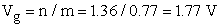and consequently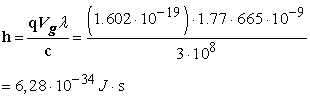A similar behaviour is obtained for all other coloured leds.

PHOTOELECTRIC EFFECT METHOD

The resulting measurements are summarized in Table 3.

Table 3. Data of Potential ArrestDownload asVeiw tableTables index

Table 3 represents the data relating to the different values of the potential shutdown. For each filter, ten measures have been taken. The students, with the help of a spreadsheet, have arranged to iterate the calculation of h as identified by the equation 5. For example, by referring to the two filters, blue and green, the relative potentials of arrest were inserted into the equation above, and by using the tabulated value of their frequencies together with the one of the electron charge, the result is:Figure 8 presents the graph on the averages course of the arrest potentials measures, related to the specific frequencies of incident radiation.Download asVeiw figureFigures index
Figure 8. The linear graph of average (on the abscissa the values of the potentials of arrest, and on the ordinates the calculated values of h

4. Discussion

For each values obtained of h has been calculated the percentage error as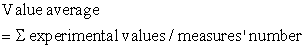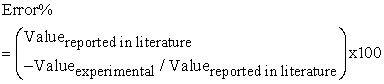The experimental value of h is compared to the one present in the scientific literature. In Table 4, h measured values are confronted.

Table 4. Comparison of the values of h calculated by using the two methodsDownload asVeiw tableTables index

For what concerns the limits connected to the two methods, it can be easily said that the major problem is related to errors in the potential measurement, which is obtained by extrapolating the linear part of the curve of one LED and the same potential arrest measurement. Since, however, the purpose of the work appears to have definitely educational aspects, what is relevant though, beyond acceptable values, is the order of magnitude of the measures, which is still respected.

5. Conclusions

Within the framework of a master on the Didactics of Science, the authors respectively a chemist and a physicist offer their students two different ways to measure the Planck’s constant: as measurement of the energy of the photons emitted by LEDs diode and the study of the photoelectric effect induced by the light emitted from a mercury lamp.

The authors, involved students by using the IBSE methodology in a series of activities of observation, exploration, construction of circuits, adjustment of a optical bench, use of computer equipment, data processing, analysis of the errors, which allowed them to acquire an experimental mode to calculate the Planck constant.

In the first method, the value of Planck's constant has been determined through current-voltage measurements. The mean value obtained is within the limits of experimental error and in good agreement with the value of literature, considering the available experimental apparatus.

The light emission of the LED starts from a value of potential (Vg) that corresponds to the voltage for which the electrons are able to recombine with “holes” of the valence band.

In the second method, the mean value is obtained by h single values measured in relation to the confrontation among all the different filters: Blue - Green, Blue – Yellow and Green – Yellow. The arrest potentials used are those of the mean values measured in Table 2. Even in this case, the mean value obtained is within the limits of experimental error and in good agreement with the value of literature, considering the available experimental apparatus.

Acknowledgements

Andrea Checchetti thanks Giovanni Orlando from I.I.S. “Eleonora Pimentel” of San Giovanni in Fiore (Italy) for his help. Alessandro Fantini thanks prof. Luigi A. Macrì for his contribution.

The authors thanks Dr. Gabriella Fantini (Italy) and prof. Donato Martano for their help throughout the translation.

The Institute of Higher Education “Eleonora Pimentel” of San Giovanni in Fiore (Italy) and the Scientific Lyceum “Enrico Fermi” of Catanzaro Lido (Italy) supported this work.

References

  Bell, T. Urhahne, D., Schanze, S. & Ploetzner, R., (2010) Collaborative Inquiry learning: Models, tools and challenges, International Journal of science education, 32(3), pp. 349-377.In article View Article  Maria Rute de Amorim e Sá Ferreira André and Paulo Sérgio de Brito André, “Classroom fundamentals: measuring the Planck constant”, online available on Science in School, http://www.scienceinschool.org/2014/issue28/planck.In article  Cloninger, T. Zhou, F. “Computer-Based Experiment for Determining Planck's constant Using LED's” The Physics Teacher. 46. (2008): 413-415.In article View Article  Raymond A. Serway “Physics for Scientists and Engineers” S.E.S. (1986): 557-560.In article  David Halliday, Robert Resnick “Fisica” C.ed. Ambrosiana (1966): 591-595.In article